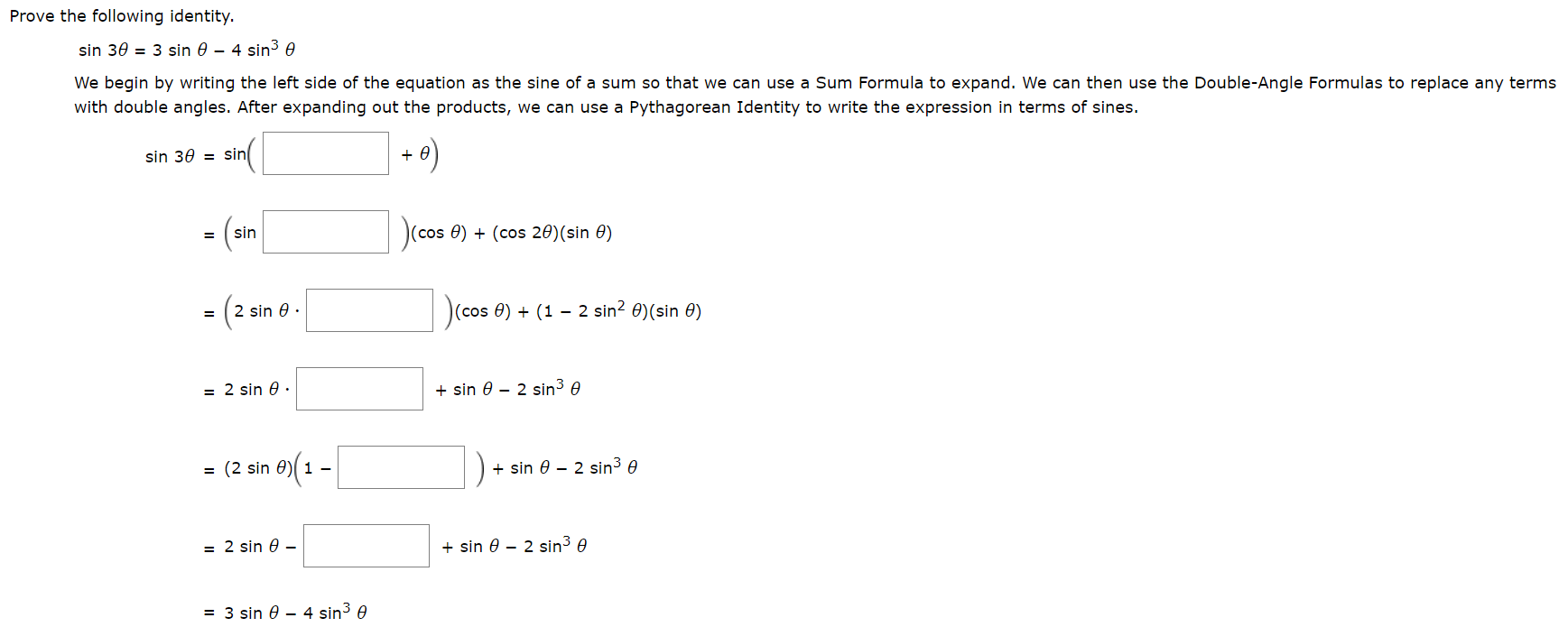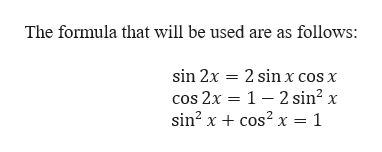# Prove the following identity3 sin e - 4 sin3 esin 30We begin by writing the left side of the equation as the sine of a sum so that we can use a Sum Formula to expand. We can then use the Double-Angle Formulas to replace any termswith double angles. After expanding out the products, we can use a Pythagorean Identity to write the expression in terms of sines.+e)sin 30= sin(am(cos e)(cos 20)(sin 0)sin(cos e) (1 - 2 sin2 e)(sin e)2 sin 0sin 0 2 sin3 e= 2 sin esin 0 2 sin3 e= (2 sin e)1 -sin 0 2 sin3 e= 2 sin e -= 3 sin 0 - 4 sin3 e

Question
47 views

photo attachedhelp_outlineImage TranscriptioncloseProve the following identity 3 sin e - 4 sin3 e sin 30 We begin by writing the left side of the equation as the sine of a sum so that we can use a Sum Formula to expand. We can then use the Double-Angle Formulas to replace any terms with double angles. After expanding out the products, we can use a Pythagorean Identity to write the expression in terms of sines. +e) sin 30= sin (am (cos e) (cos 20)(sin 0) sin (cos e) (1 - 2 sin2 e)(sin e) 2 sin 0 sin 0 2 sin3 e = 2 sin e sin 0 2 sin3 e = (2 sin e)1 - sin 0 2 sin3 e = 2 sin e - = 3 sin 0 - 4 sin3 e fullscreen
check_circle

Step 1

To prove:

Step 2

Note:

...help_outlineImage TranscriptioncloseThe formula that will be used are as follows: sin 2x 2 sin x cos x cos 2x 1 2 sin2 : sin2 xcos2 x = 1 fullscreen

### Want to see the full answer?

See Solution

#### Want to see this answer and more?

Solutions are written by subject experts who are available 24/7. Questions are typically answered within 1 hour.*

See Solution
*Response times may vary by subject and question.
Tagged in

### Trigonometric Ratios